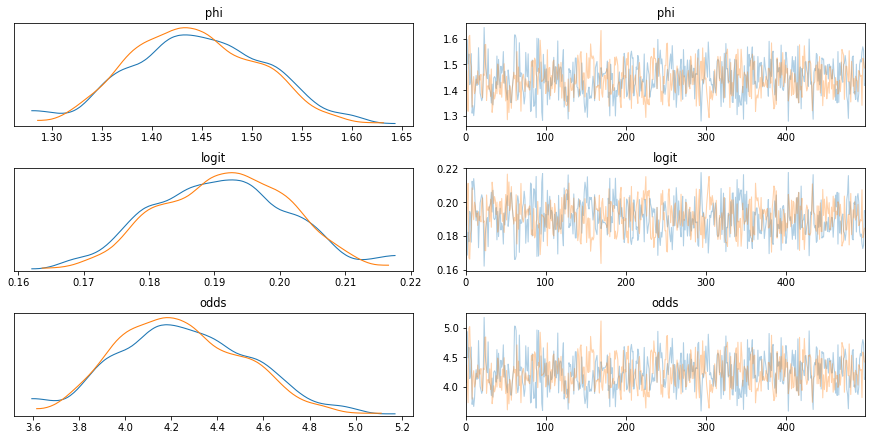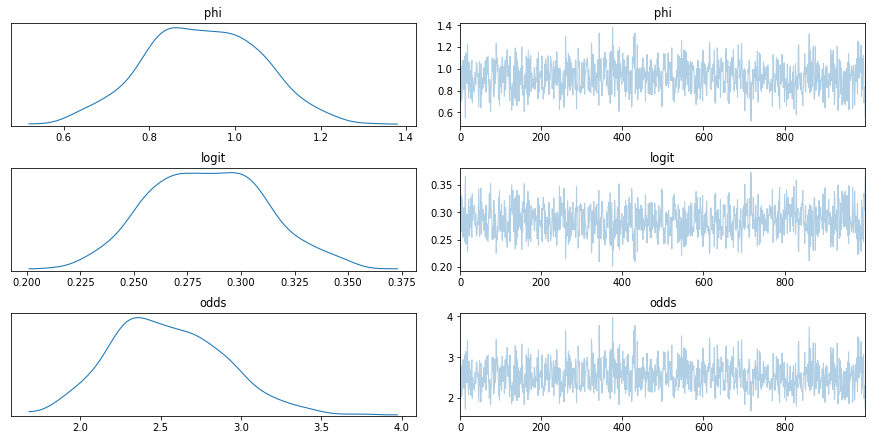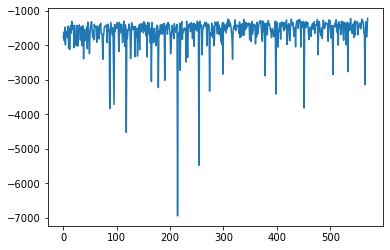In :
import pymc3 as pm
import theano
import numpy as np
%matplotlib inline
import matplotlib.pyplot as plt

WARNING (theano.configdefaults): install mkl with conda install mkl-service: No module named 'mkl'


# Generate some data:¶

This is for a hierarchical model to measure the proportion of some event. It generates about 1 to 10 samples of binary 0 or 1 measurements, where 1's (the event) occur 20% of the time.

There will be some variability. So the goal is to measure the proportion of 1's while modeling each instance individually and sharing overall mean probability --> i.e. a hierarchical model

We thus expect the probability to be ~20%, and/or the odds to be ~1/4.

### The data:¶

In :
#generate data:
#Creating a synthetic dataset:
data = list()

#probability of 0 or 1:
probs = [0.8, 0.2]
#take measurements for 500 instances:
for j in range(500):

observations = list()
#all instances must have at least one measurement:
observations.append(np.random.choice([0,1], p=probs))
#now take several more measurements for this instance:
while np.random.uniform(0,1)>0.2:
observations.append(np.random.choice([0,1], p=probs))
data.append(observations)

#print a sample to see what the measuments are like
for i in range(5):
print(f'Sample {i}: {data[i]}')

#parse for input to pymc3:
num_measurements = [len(i) for i in data]
num_events = [sum(i) for i in data]
N = len(data)

Sample 0: 
Sample 1: 
Sample 2: 
Sample 3: [0, 0, 0, 0, 0, 0, 0, 0, 0]
Sample 4: [0, 0, 0, 0, 0, 1, 0]


### The model:¶

The group parameter (phi) is measuring the log probability of the event. This can be transformed into a probability by a logit (logit) and then to odds (odds). The instance parameters are beta distributions, with parameters 'a' and 'b' determined by the odds, i.e. odds of 1:odds.

Likelihood is measured by a binomial, where you use the probability determined by the priors above along with the number of measurements and the sum of measurements for each instance as the observations.

In :
#pymc3 gear:
with pm.Model() as model:
#group parameter:
phi = pm.Normal('phi', mu=0, sigma=1.0)
#probability:
logit = pm.Deterministic('logit', 1 / (1 + np.exp(phi)))
odds = pm.Deterministic('odds', (1-logit)/logit)

#instance parameters:
thetas = pm.Beta('thetas', alpha=1, beta=odds, shape=N)

#likelihood:
y = pm.Binomial('y', n=num_measurements, p=thetas, observed=num_events)


### Short NUTS run works well:¶

See that logit encapsulates the expected 20%

In :
with model:
trace = pm.sample(draws=500, tune=500, chains=2,
target_accept=0.9)

pm.traceplot(trace, var_names=['phi', 'logit','odds'])

Auto-assigning NUTS sampler...
Multiprocess sampling (2 chains in 2 jobs)
NUTS: [thetas, phi]

100.00% [2000/2000 00:06<00:00 Sampling 2 chains, 0 divergences]
/Users/lmar3213/miniconda3/envs/ppl/lib/python3.8/site-packages/arviz/plots/backends/matplotlib/distplot.py:36: UserWarning: Argument backend_kwargs has not effect in matplotlib.plot_distSupplied value won't be used
warnings.warn(
/Users/lmar3213/miniconda3/envs/ppl/lib/python3.8/site-packages/arviz/plots/backends/matplotlib/distplot.py:36: UserWarning: Argument backend_kwargs has not effect in matplotlib.plot_distSupplied value won't be used
warnings.warn(
/Users/lmar3213/miniconda3/envs/ppl/lib/python3.8/site-packages/arviz/plots/backends/matplotlib/distplot.py:36: UserWarning: Argument backend_kwargs has not effect in matplotlib.plot_distSupplied value won't be used
warnings.warn(

Out:
array([[<matplotlib.axes._subplots.AxesSubplot object at 0x11e668580>,
<matplotlib.axes._subplots.AxesSubplot object at 0x11e660700>],
[<matplotlib.axes._subplots.AxesSubplot object at 0x11e756b50>,
<matplotlib.axes._subplots.AxesSubplot object at 0x11e7849a0>],
[<matplotlib.axes._subplots.AxesSubplot object at 0x11e7b1a00>,
<matplotlib.axes._subplots.AxesSubplot object at 0x11e7dca60>]],
dtype=object)### ADVI run performs not so well:¶

logit does has basically no density around 20%

In :
with model:
mean_field = pm.fit(n=10000,method=inference)

trace = mean_field.sample(1000)
pm.traceplot(trace, var_names=['phi', 'logit','odds'])

100.00% [10000/10000 01:04<00:00 Average Loss = 681.1]
/Users/lmar3213/miniconda3/envs/ppl/lib/python3.8/site-packages/theano/tensor/subtensor.py:2339: FutureWarning: Using a non-tuple sequence for multidimensional indexing is deprecated; use arr[tuple(seq)] instead of arr[seq]. In the future this will be interpreted as an array index, arr[np.array(seq)], which will result either in an error or a different result.
out[inputs[2:]] = inputs
/Users/lmar3213/miniconda3/envs/ppl/lib/python3.8/site-packages/theano/tensor/subtensor.py:2197: FutureWarning: Using a non-tuple sequence for multidimensional indexing is deprecated; use arr[tuple(seq)] instead of arr[seq]. In the future this will be interpreted as an array index, arr[np.array(seq)], which will result either in an error or a different result.
rval = inputs.__getitem__(inputs[1:])
/Users/lmar3213/miniconda3/envs/ppl/lib/python3.8/site-packages/theano/tensor/basic.py:6611: FutureWarning: Using a non-tuple sequence for multidimensional indexing is deprecated; use arr[tuple(seq)] instead of arr[seq]. In the future this will be interpreted as an array index, arr[np.array(seq)], which will result either in an error or a different result.
result[diagonal_slice] = x
Finished [100%]: Average Loss = 680.96
/Users/lmar3213/miniconda3/envs/ppl/lib/python3.8/site-packages/arviz/plots/backends/matplotlib/distplot.py:36: UserWarning: Argument backend_kwargs has not effect in matplotlib.plot_distSupplied value won't be used
warnings.warn(
/Users/lmar3213/miniconda3/envs/ppl/lib/python3.8/site-packages/arviz/plots/backends/matplotlib/distplot.py:36: UserWarning: Argument backend_kwargs has not effect in matplotlib.plot_distSupplied value won't be used
warnings.warn(
/Users/lmar3213/miniconda3/envs/ppl/lib/python3.8/site-packages/arviz/plots/backends/matplotlib/distplot.py:36: UserWarning: Argument backend_kwargs has not effect in matplotlib.plot_distSupplied value won't be used
warnings.warn(

Out:
array([[<matplotlib.axes._subplots.AxesSubplot object at 0x127454190>,
<matplotlib.axes._subplots.AxesSubplot object at 0x1274230a0>],
[<matplotlib.axes._subplots.AxesSubplot object at 0x12816f0a0>,
<matplotlib.axes._subplots.AxesSubplot object at 0x128210100>],
[<matplotlib.axes._subplots.AxesSubplot object at 0x12823e160>,
<matplotlib.axes._subplots.AxesSubplot object at 0x12826b310>]],
dtype=object)In :
#plot convergence:
plt.plot(-inference.hist)

Out:
[<matplotlib.lines.Line2D at 0x1249002e0>]In [ ]: